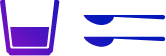# Liter to Quart - Quart to Liters

## Convert Quarts to Liters (qt to l) and Liters to Quarts (l to qt)

Home

Converter

Liter - Quart Converter

You can input in any of the fields and get equivalent values.
=

## Quarts and Liters – An Overview• ### Quarts and liters are both units of volume

. However, they are used in different measurement systems.
• Quarts are used in the imperial system of units, while liters are used in the metric system of units.
• ### A quart is slightly larger than a liter. One quart is equal to 1.05669 liters.

• Quarts are commonly used in the United States for measuring liquids, such as milk, water, and gasoline.
• Liters are commonly used in the rest of the world for measuring liquids and other things, such as food, fuel, and chemicals.
• Liters are also used to measure the volume of liquids in containers, such as bottles and jugs. For example, a 2-liter bottle of soda contains 2 liters of soda.
• Liters are also used to measure the volume of solids, such as sand and gravel. For example, a cubic meter of sand has a volume of 1,000 liters.
Here are some interesting facts about quarts and liters:
• The word "quart" comes from the Latin word "quartus," which means "fourth." This is because a quart is one fourth of a gallon.
• The word "liter" comes from the Greek word "litron," which means "unit of weight."
• The metric system was developed in France in the late 18th century. The imperial system was developed in the United Kingdom in the early 19th century.
• The metric system is the most widely used measurement system in the world.
• The United States is one of the few countries that still uses the imperial system.
Differences between liters and quarts:
• A quart is slightly larger than a liter. One quart is equal to 1.05669 liters.
• Liters are used in the metric system, which is the most widely used measurement system in the world.
• Quarts are used in the imperial system, which is less common than the metric system.

## Liter to Quart ConvertersWhere liters are used:
• Liters are used in most countries in the world to measure liquids and other things, such as food, fuel, and chemicals.
• Liters are also used to measure the volume of liquids in containers, such as bottles and jugs.
• Liters are also used to measure the volume of solids, such as sand and gravel.
Where quarts are used:
• Quarts are commonly used in the United States for measuring liquids, such as milk, water, and gasoline.
• Quarts are also used in some other countries, such as the United Kingdom and Canada, but they are less common than liters.
How liters and quarts are used in different contexts:
• When you buy a bottle of soda at the store, it is typically labeled in liters.
• When you go to the gas station, you pump gasoline into your car in gallons. However, gallons are also divided into quarts.
• When you cook, you might measure ingredients like flour and sugar in cups. However, cups can also be converted to liters or quarts.
• ### Liter to quart converter is a useful tool for anyone who needs to convert between the two units of volume

. It is accurate, convenient, and versatile.
Examples of how a liter to quart converter can be used:
• A baker might use a liter to quart converter to convert the measurements in a recipe from the metric system to the imperial system.
• For a traveler, a liter to quart converter is useful to convert the measurements on a gasoline pump in a foreign country.
• A cook can use a liter to quart converter to measure ingredients for a recipe when they only have measuring cups that are marked in liters or quarts.

Note: The above content has inputs from Bard, the AI model from Google

## Liter to Quart Conversion (l to qt) Table## Quarts to Liters converter(qt to l) table### FAQ on Liter and Quart Conversion